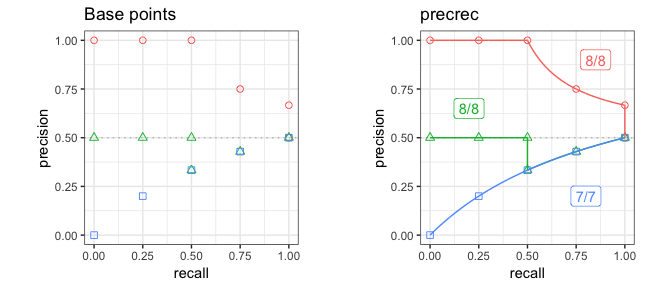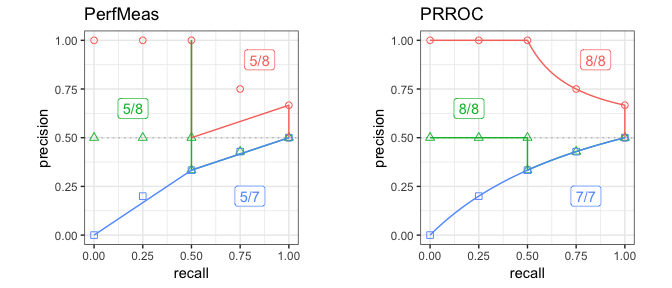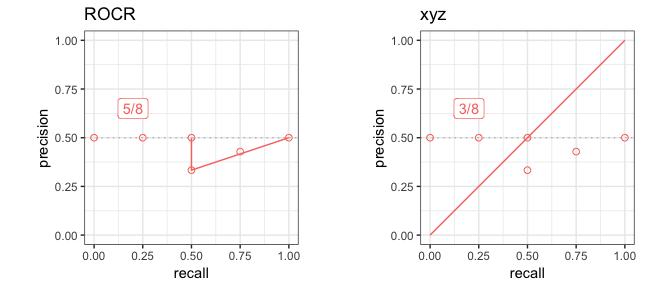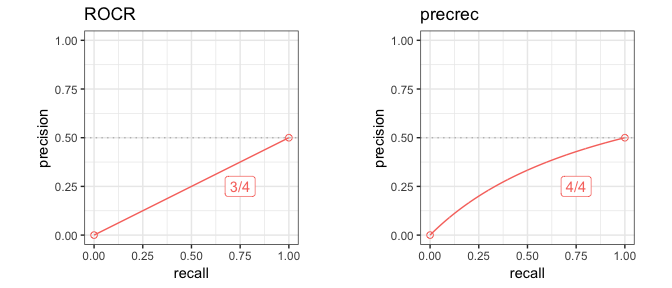Introduction to prcbench

2019-03-05

The prcbench package is a testing workbench for evaluating Precision-Recall curves.

1. Tool interface

The prcbench package provides predefined interfaces for the following five tools that calculate Precision-Recall curves.

ROCR R Tool web site, CRAN
AUCCalculator Java Tool web site
PerfMeas R CRAN
PRROC R CRAN
precrec R Tool web site, CRAN

Create a tool set

The create_toolset function generates a tool set with a combination of the five tools.

library(prcbench)

## A single tool
toolsetA <- create_toolset("ROCR")

## Multiple tools
toolsetB <- create_toolset(c("PerfMeas", "PRROC"))

## Tool sets can be manually combined to a single set
toolsetAB <- c(toolsetA, toolsetB)

The create_toolset function accepts partially matched tool names. Tool names may also be case-insensitive.

library(prcbench)

## A single tool - lower case
toolsetA2 <- create_toolset("rocr")

## Multiple tools - lower case and partially matched
toolsetB2 <- create_toolset(c("perf", "prr"))

Use predefined tool sets

The create_toolset function takes two additional arguments - calc_auc and store_res. The calc_auc argument makes tools calculate and retrieve the AUC score, and the store_res argument forces tools to calculate and store the curve values.

The following six tool sets are predefined with a different combination of tools and their argument values.

Set name Tools calc_auc store_res
def5 ROCR, AUCCalculator, PerfMeas, PRROC, precrec TRUE TRUE
auc5 ROCR, AUCCalculator, PerfMeas, PRROC, precrec TRUE FALSE
crv5 ROCR, AUCCalculator, PerfMeas, PRROC, precrec FALSE TRUE
def4 ROCR, AUCCalculator, PerfMeas, precrec TRUE TRUE
auc4 ROCR, AUCCalculator, PerfMeas, precrec TRUE FALSE
crv4 ROCR, AUCCalculator, PerfMeas, precrec FALSE TRUE
## Use 'set_names'
toolsetC <- create_toolset(set_names = "auc5")

## Multiple sets are automatically combined to a single set
toolsetD <- create_toolset(set_names = c("auc5", "crv4"))

2. Test data interface

The prcbench package provides two different types of test data. The first one is for benchmarking, and the second one is for evaluating the accuracy of Precision-Recall curves.

The create_testset function offers both types of test data by setting the first argument either as “bench” or “curve”.

Create a test dataset for benchmarking

The create_testset function uses a naming convention for randomly generated data for benchmarking. The format is a prefix (‘b’ or ‘i’) followed by the number of dataset. The prefix ‘b’ indicates a balanced dataset, whereas ‘i’ indicates an imbalanced dataset. The number can be used with a suffix ‘k’ or ‘m’, indicating respectively 1000 or 1 million.

## A balanced data set with 50 positives and 50 negatives
testset1A <- create_testset("bench", "b100")

## An imbalanced data set with 2500 positives and 7500 negatives
testset1B <- create_testset("bench", "i10k")

## Test data sets can be manually combined to a single set
testset1AB <- c(testset1A, testset1B)

## Multiple sets are automatically combined to a single set
testset1C <- create_testset("bench", c("i10", "b10"))

Create a test dataset for curve evaluation

The create_testset function takes predefined set names for curve evaluation. These data sets contain pre-calculated precision and recall values. The pre-calculated values must be correct so that they can be compared with the results of specified tools.

The following three test sets are currently available.

• c1
• c2
• c3
## C1 test set
testset2A <- create_testset("curve", "c1")

## C2 test set
testset2B <- create_testset("curve", "c2")

## Test data sets can be manually combined to a single set
testset2AB <- c(testset2A, testset2B)

## Multiple sets are automatically combined to a single set
testset2C <- create_testset("curve", c("c1", "c2"))

3. Benchmarking

The run_benchmark function internally calls the microbenchmark function provided by the microbenchmark package. It takes a test set and a tool set and returns the result of microbenchmark.

## Run microbenchmark for aut5 on b10
testset <- create_testset("bench", "b10")
toolset <- create_toolset(set_names = "auc5")
res <- run_benchmark(testset, toolset)
res
##   testset toolset      toolname   min    lq mean median     uq max neval
## 1     b10    auc5          ROCR 1.598 1.607 19.4  1.669 12.470  80     5
## 2     b10    auc5 AUCCalculator 3.069 3.594 10.1  4.004  5.932  34     5
## 3     b10    auc5      PerfMeas 0.065 0.066 78.2  0.072  0.092 391     5
## 4     b10    auc5         PRROC 0.169 0.173  2.6  0.181  0.213  12     5
## 5     b10    auc5       precrec 4.133 4.289 23.0  4.614  5.757  96     5

4. Evaluation of Precision-Recall curves

The run_evalcurve function evaluates Precision-Recall curves with the following five test cases.

Test case Description
x_range Evaluate the range of recall values
y_range Evaluate the range of precision values
fpoint Check the first point
int_pts Check the intermediate points
epoint Check the end point

Evaluation scores

The run_evalcurve function calculates the scores of the test cases and summarizes them to a data frame.

## Evaluate Precision-Recall curves for ROCR and precrec with c1 test set
testset <- create_testset("curve", "c1")
toolset <- create_toolset(c("ROCR", "precrec"))
scores <- run_evalcurve(testset, toolset)
scores
##   testset toolset toolname score
## 1      c1    ROCR     ROCR   5/8
## 2      c1 precrec  precrec   8/8

The result of each test case can be displayed by specifying data_type = all of the print function.

## Print all results
print(scores, data_type = "all")
##    testset toolset toolname testitem testcat success total
## 1       c1    ROCR     ROCR  x_range      Rg       1     1
## 2       c1    ROCR     ROCR  y_range      Rg       1     1
## 3       c1    ROCR     ROCR   fpoint      SE       0     1
## 4       c1    ROCR     ROCR   intpts      Ip       2     4
## 5       c1    ROCR     ROCR   epoint      SE       1     1
## 6       c1 precrec  precrec  x_range      Rg       1     1
## 7       c1 precrec  precrec  y_range      Rg       1     1
## 8       c1 precrec  precrec   fpoint      SE       1     1
## 9       c1 precrec  precrec   intpts      Ip       4     4
## 10      c1 precrec  precrec   epoint      SE       1     1

Visualization of the result

The autoplot shows a plot with the result of the run_evalcurve function.

## ggplot2 is necessary to use autoplot
library(ggplot2)

## Plot base points and the result of precrec on c1, c2, and c3 test sets
testset <- create_testset("curve", c("c1", "c2", "c3"))
toolset <- create_toolset("precrec")
scores1 <- run_evalcurve(testset, toolset)
autoplot(scores1)

## Plot the results of PerfMeas and PRROC on c1, c2, and c3 test sets
toolset <- create_toolset(c("PerfMeas", "PRROC"))
scores2 <- run_evalcurve(testset, toolset)
autoplot(scores2, base_plot = FALSE)5. Create a user-defined tool interface

In addition to the predefined five tools, users can add new tool interfaces for their own tools to run benchmarking and curve evaluation. The create_usrtool function takes a name of the tool and a function for calculating a Precision-Recall curve.

## Create a new tool set for 'xyz'
toolname <- "xyz"
calcfunc <- create_example_func()
toolsetU <- create_usrtool(toolname, calcfunc)

## User-defined tools can be combined with predefined tools
toolsetA <- create_toolset("ROCR")
toolsetU2 <- c(toolsetA, toolsetU)

Like the predefined tool sets, user-defined tool sets can be used for both run_benchmark and run_evalcurve.

## Curve evaluation
testset3 <- create_testset("curve", "c2")
scores3 <- run_evalcurve(testset3, toolsetU2)
autoplot(scores3, base_plot = FALSE)The format of the function for calculating a Precision-Recall curve

The create_example_func function creates an example for the second argument of the create_usrtool function. The actual function should also take a testset generated by the create_testset function and returns a list with three elements - x, y, and auc.

## Show an example of the second argument
calcfunc <- create_example_func()
print(calcfunc)
## function (single_testset)
## {
##     scores <- single_testset$get_scores() ## labels <- single_testset$get_labels()
##     pred <- list(x = seq(0, 1, 1/length(scores)), y = seq(0,
##         1, 1/length(scores)), auc = 0.5)
## }
## <bytecode: 0x7fa60e37c078>
## <environment: 0x7fa610229bb8>

The create_testset function produces a testset as either TestDataB or TestDataC object. See the help files of the R6 classes - help(TestDataB) and help(TestDataC) - for the methods that can be used with the Precision-Recall calculation.

6. Create a user-defined test data interface

The prcbench package also supports user-defined test data interfaces. The create_usrdata function creates two types of test datasets.

Create a user-defined test dataset for benchmarking

The first argument of the create_usrdata function should be “bench” to create a test data for benchmarking. Additionally, scores and labels are required.

## Create a test dataset 'b5' for benchmarking
testsetB <- create_usrdata("bench", scores = c(0.1, 0.2), labels = c(1, 0),
tsname = "b5")

It can be used in the same way as the predefined test datasets for benchmarking.

## Run microbenchmark for ROCR and precrec on a predefined test dataset
toolset <- create_toolset(c("ROCR", "precrec"))
res <- run_benchmark(testsetB, toolset)
res
##   testset toolset toolname min  lq mean median  uq max neval
## 1      b5    ROCR     ROCR 2.1 2.2  2.4    2.3 2.4 2.9     5
## 2      b5 precrec  precrec 4.4 4.4  4.7    4.6 4.9 5.5     5

Create a user-defined test dataset for curve evaluation

The first argument of the create_usrdata function should be “curve” to create a test dataset for curve evaluation. Scores and labels as well as pre-calculated recall and precision values are required. These pre-calculated values are used to compare with the corresponding values predicted by the specified tools.

## Create a test dataset 'c5' for benchmarking
testsetC <- create_usrdata("curve", scores = c(0.1, 0.2), labels = c(1, 0),
tsname = "c5", base_x = c(0.0, 1.0),
base_y = c(0.0, 0.5))

It can be used in the same way as the predefined test datasets for curve evaluation.

## Run curve evaluation for ROCR and precrec on a predefined test dataset
toolset2 <- create_toolset(c("ROCR", "precrec"))
scores2 <- run_evalcurve(testsetC, toolset2)
autoplot(scores2, base_plot = FALSE)